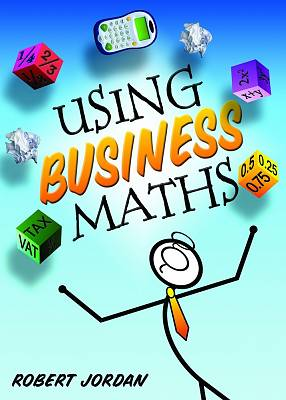New student-friendly textbook introducing common calculations encountered regularly in both personal and business scenarios. * Presents calculations and problems in a clear and logical manner using humour and illustrations to aid understanding. * Positions the concepts of maths and calculations in the real world and demonstrates the importance of maths in everyday life. * Demonstrates how to use a calculator accurately to perform extended calculations, as well as how to perform simple calculations without a calculator. * Clearly explains percentages, law of indices, rules of logarithms, simple and compound interest, payroll and associated taxes, VAT, net profit and loss and algebra, including quadratic equations. * Illustrates how to mathematise scenarios, make an initial model, decide on appropriate mathematical techniques and the tools to apply them, examine relationships and assumptions, make adjustments and present results and conclusions. * Includes sample questions and answers along with graded end-of-chapter questions. Specifically written for the revised FETAC Level 4 modules Business Calculations (4N2279) and Functional Mathematics (4N2138)

240 pages.

ISBN: 9780717157150

€ 22.99

In Stock.

Usually despatched in 2-5 working days.

Date of Publication: 21/05/2013Paperback

Using Business Maths also appears in these Categories: